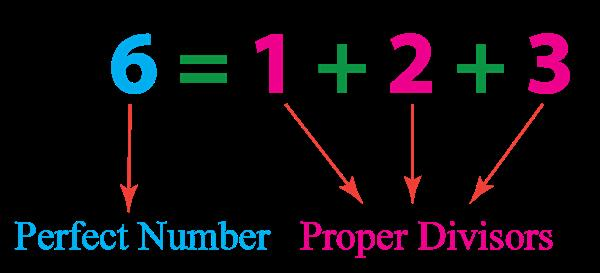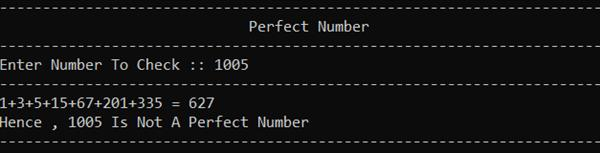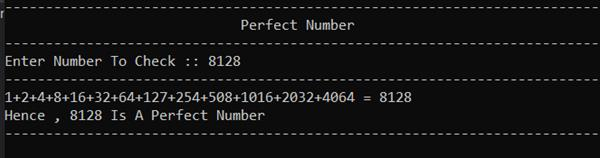Loading, please wait...# Program To Check Whether A Number Is A Perfect Number Or Not

In this blog, we will create a program in C# to check if an entered number is a perfect number or not.

## Introduction

Perfect numbers are positive integers that are equal to the sum of its factors except for the number itself. In other words, perfect numbers are the positive integers which are the sum of their proper divisors. The smallest perfect number is 6, which is the sum of its proper divisors: 1, 2, and 3.Program to check perfect number

``````using System;
using System.Collections.Generic;
using System.Linq;
using System.Text;

namespace PerfectNumber
{
class Program
{
static void Main(string[] args)
{
int number, sum = 0;
string divisors = "";
Console.WriteLine("---------------------------------------------------------------");
Console.WriteLine("                   Perfect Number                              ");
Console.WriteLine("----------------------------------------------------------------");
Console.Write("Enter Number To Check :: ");

for (int i = 1; i < number; i++)
{
if (number % i == 0)
{
sum += i;
divisors += i.ToString() + "+";
}
}
divisors = divisors.Remove(divisors.Length - 1, 1);
Console.WriteLine("---------------------------------------------------------------");
Console.WriteLine(divisors + " = " + sum);
if (sum == number)
{
Console.WriteLine("Hence , " + number + " Is A Perfect Number");
}
else
Console.WriteLine("Hence , " + number + " Is Not A Perfect Number");

Console.WriteLine("---------------------------------------------------------------");
}
}
}  ``````

Code Explanation

• First, we define three variables number type of integer, sum type of integer, and divisors type of string. We use numbers to get the input of a user, a sum to get the sum of the divisor, and divisors to add all divisors of the numbers in one string.
• Then we get input from the user and pass it to the number variable by converting it to an integer.
• Then we iterate a loop from 1 (one) to number – 1.
• In the loop, we module number by iterate variable I. If the remainder is 0 then add that number in sum and concat value of I in our variable divisors with plus (+) sign.
• After the loop, we remove the last one character from the divisors variable because the extra one + is also concat in the string. Here we just show this as all divisors sum is equal to sum for better user understanding.
• Next, we check if the sum is equal to the user’s input. If yes, then it shows a message that the entered number is a perfect number. Otherwise, the entered number is not a perfect number.
• Then, we use the Console.ReadKey() method to read any key from the console. By entering this line of code, the program will wait and not exit immediately. The program will wait for the user to enter any key before finally exiting. If you don't include this statement in the code, the program will exit as soon as it is run.
OutputI hope you find this blog helpful. If you get any help from this blog, please share it with your friends.